This lesson explains mainly PID parameters, angle loop , speed loop , rotation loop

### Debugging Guide

1. Angle loop parameters
2. speed loop parameters
3. rotating ring parameters

The power to keep the car upright and running is derived from the two wheels of the car.Wheel rotation is driven by two DC motors.Therefore, the car as a control object, the control input is the rotation speed of the two motors from the control point of view, trolley motion control task can be decomposed into the following three basic control tasks:

1: Control car balance:Keeping the state of upright and balance of the car by controlling the forward and reverse movement of the two motors;

2. Control car speed:Car speed control is achieved by adjusting the positional deviation of the car,in the end it actually evolved to control the speed of the motor to achieve wheel speed control;

3. Control the direction of the car:The steering control of the car is achieved by controlling the rotational differential between the two motors.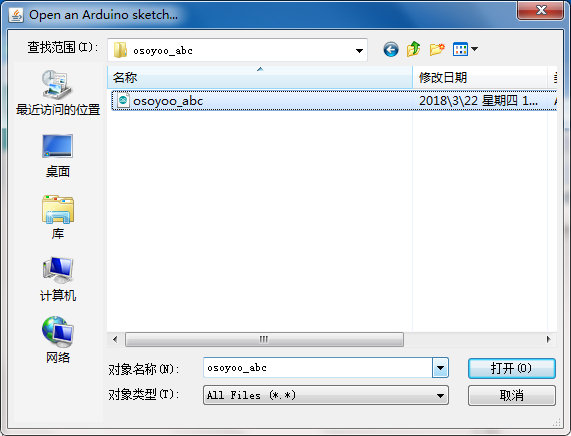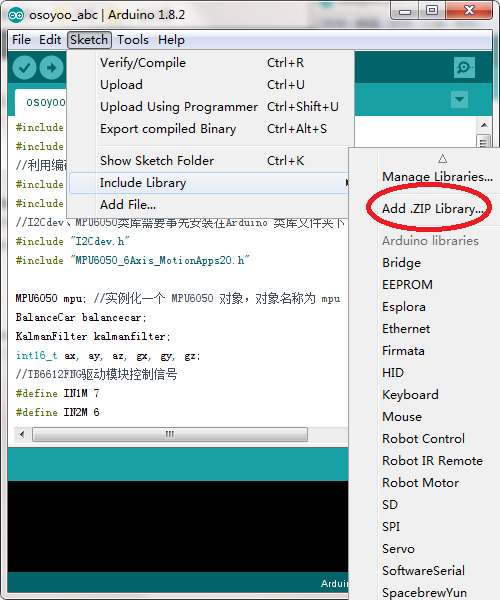The second method: unzipping files of balance libraries directly,Copying and pasting the file that under the directory directly on the libary directory that under the arduino installation path.### Commissioning guide

The control of the balance, speed, and direction of the car uses angle, speed, and direction rings,respectively.Debugging parameters of PID is a long process.Each link has a certain degree of influence with each other. Therefore, it needs to be debugged patiently to find a set of optimal parameters.

#### 1:Angle parameter

The balance car angle uses a PD (proportional derivative) controller.In debugging, we need to adjust the values of Kp and Kd,make the car have a good speed and stability.The parameter used for the calculation of the angle is the tilt angle of the car.Kp is the coefficient of inclination of the car,Kd is the coefficient of angular velocity,it is used to reduce the over adjustment by the proportional adjustment. It is according to the sequence :first P (proportional), after D (differential).

(1) Determine the size of Kp

The principle of determining parameters: Kp keeps increasing until there is a large amount of low frequency jitter.

1. Example: Set Kp=10, we can see the car has a balanced trend,however, it will still fall to one side more quickly.Obviously the response is too slow.
2. Set Kp=20. At this time we can see that the car has a balance trend,and the response has accelerated,but the response is still not enough to keep the car balanced.
3. Set Kp=40, this time we can see that the response of the car has been significantly accelerated, and when pushing the car back and forth,there will be a large amount of low frequency jitter, it Explains that the Kp value is large enough at this time and needs to increase differential control to weaken Kp control,suppress low frequency jitter.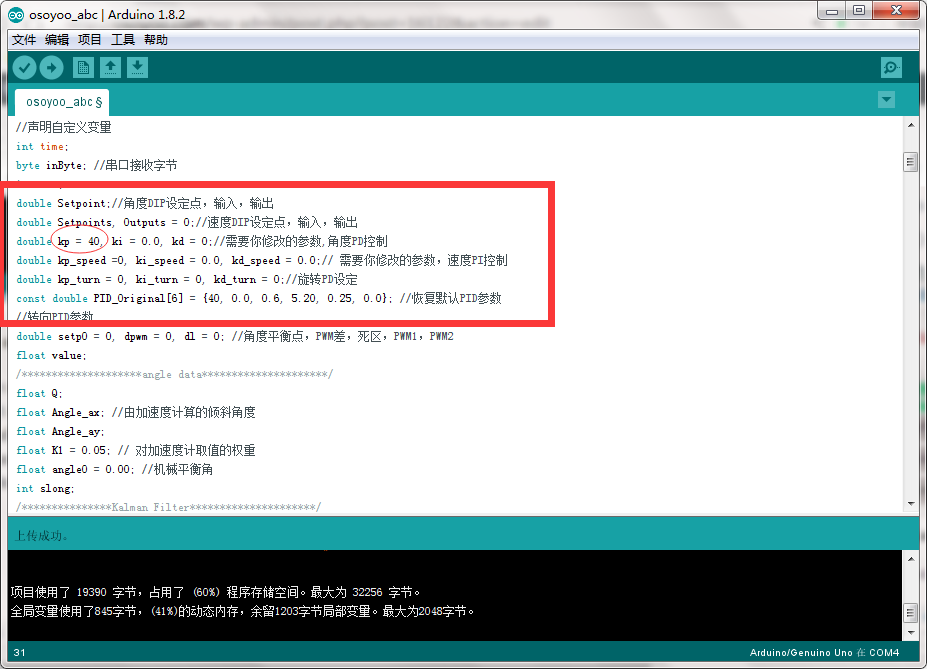(2) Determine the size of Kd

The principle of determining parameters:Kp keeps increasing until high frequency jitter occurs.

1. Example:Set Kd=0.3,at this time we can see that large amplitude low-frequency jitter has been largely eliminated.
2. Set Kd=0.5, we can see the overall performance is in a better state.
3. Set Kd=0.8, we can see that the car starts to shake at high frequency (Note: Long-time high-frequency jitter can damage the drive and the motor).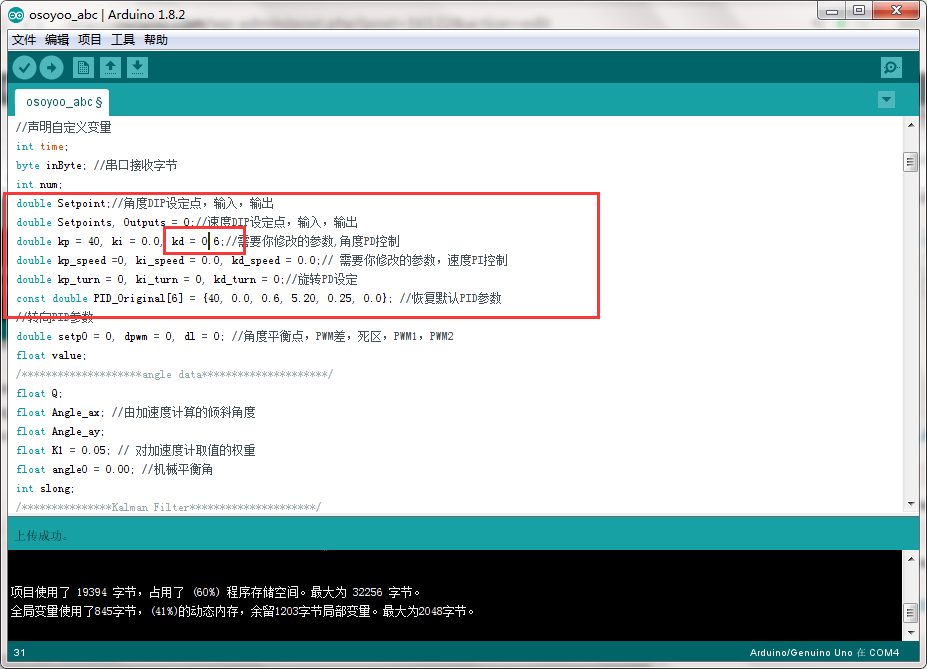We can sure that Kp=40 and Kd=0.6are the maximum parameters of P and D ,we try not to exceed these two values in the later debugging process ,decrease the value properly,you can see that the car after adjustment is smoother.However, it is still unable to maintain a long period of erection,after standing for a short period of time, it will accelerate in one direction,so we can add speed loop to get better performance,but the angle loop is very difficult for the car to keep a good upright effect.At this point, the upright debugging part has come to an end.

#### 2, speed parameters

The speed loop controls the speed of the car.On the one hand, the car is more stable when it is stationary, the car will not fall sideways,on the other hand, the car can be driven upright.The speed loop uses a PI (Proportional Integral) controller.The parameter used to calculate the speed loop is the speed of the car.It is necessary to set the parameters of upright before adjusting the speed .Here we set Kp=40 and Ki=0.6,first adjust I (integral) and then adjust P (proportion).

1.Determine the size of the Ki_speed value

1. Determine the principle of the parameters: adjusting the size of Ki_speed until the car can quickly stabilize in a fixed position.
2. Setting Ki_speed=0.1. at this time we can see that the car has a fixed position. Even if the car is pushed,it can still return to its original position.
3. setting Ki_speed=0.4, we can see that the car has faster speed after adjustment, but the car can over adjust easily and creates unnecessary shocks.Then add Kp_speed to stabilize the car’s position.2. Determine the size of the Kp_speed value The principle of determining the parameters: Kp_speed keeps increasing until the cart can stay upright and not easily fall down.

1. Example: Set Kp_speed=1.0. At this time we can see that the car will adjust by pushing back and forth within a certain distance. Although it is still not stationary completely ,but it has been able to stand upright for a long time,it can be seen that the speed ring is working.
2. Setting Kp_speed=2.5. At this time we can see that the speed of the car can be basically stable. however, pushing with one hand still falls to one side, and the anti-interference ability is poor.
3. Setting Kp_speed=4.0. At this time we can see that the response speed of the car is faster and the anti-interference ability is strengthened.When pushing by hand, the speed of the car can be quickly reduced.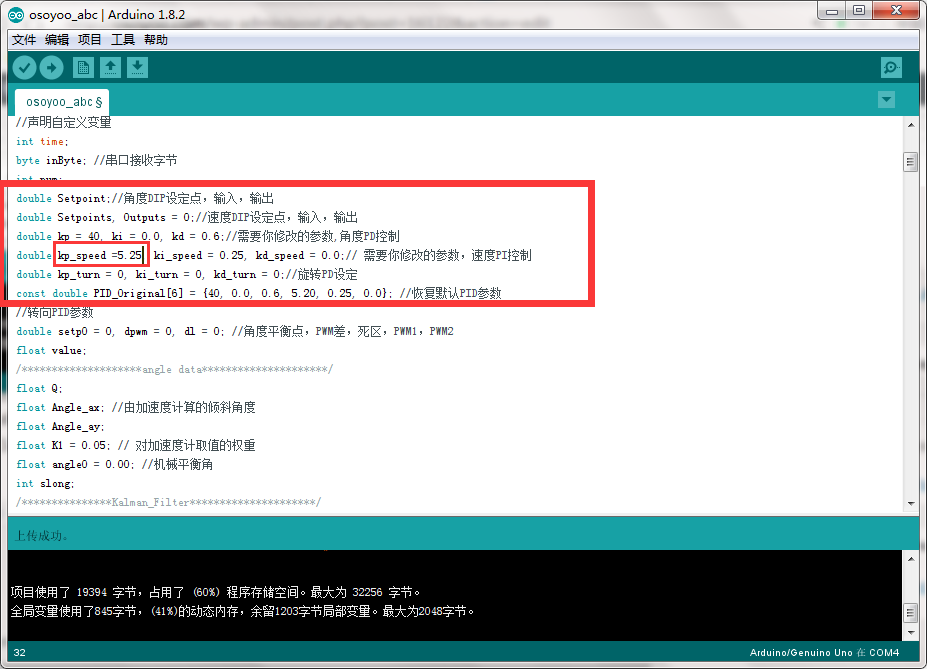Therefore, we set Ki_speed=0.25,Kp_speed=5.20,this is just a rough demonstration of the parameter debugging process,not the optimal parameter,the size of the parameters can be further fine-tuned according to the actual situation.

#### 3, direction parameter

After the adjustment of the upright ring and the speed ring,the car has been able to remain stable and upright,due to the actual deviation of the left and right motors,it is necessary to introduce a directional ring to control the direction of going straight of the car to prevent the car from yawing.

• In addition, the direction ring is also used for the speed adjustment of the car turning.Kp_turn is only used to change the speed ratio of the car turning. The larger the value is, the faster the car turns, and generally it does not need to change its size.Kd_turn is the coefficient of the Z-axis angular velocity.When the car does not go straight,the deviation of the cart can be corrected by adjusting the size of Kp_turn.
• When the value is too large, it will cause the car to oscillate around,and do not set the value too large when adjusting.
• When debugging ,the car can refer to a straight line,control the car straight, adjust the Kd_turn size until the car does not deviate from the straight line.

### summary

The above method is the static parameter adjustment method of the car.You can get the parameters of making car stand upright.If the car is in poor state of upright,the parameters need to be fine-tuned again to make the car have better state when working.In general, the proportional parameters affect the adjustment speed of the car ,Differential parameters affect the stability of the car;The integration parameters are related to the accuracy of the adjustment.The following figure shows the optimal parameters for all parameter adjustments.Differential parameters affect the stability of the car,the integration parameters are related to the accuracy of the adjustment.The following figure shows the optimal parameters for all parameter adjustments.The following describes the weight adjustment of the balance car’s load.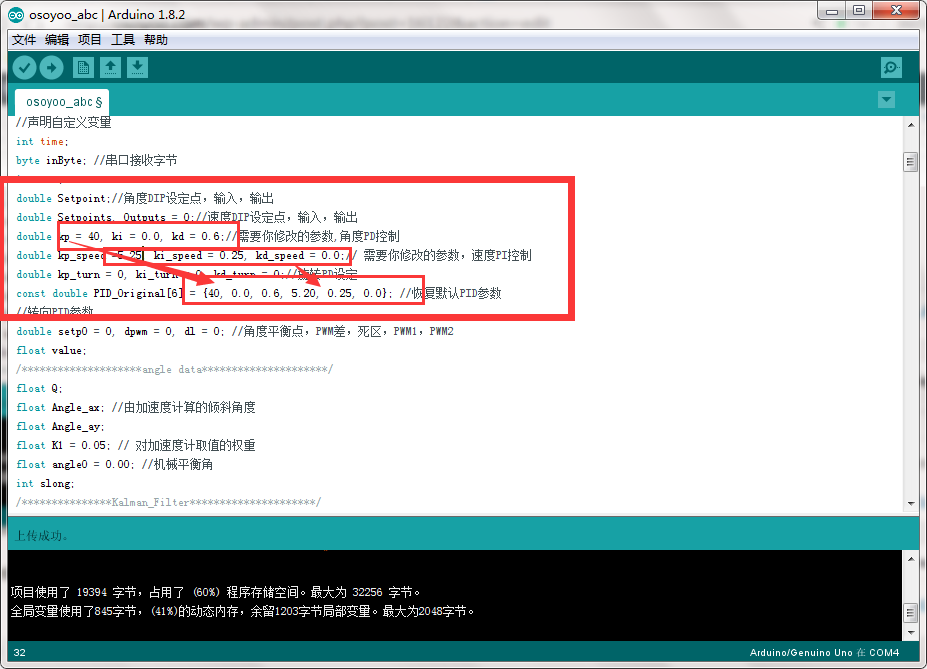The upper limit of the weight of the balance car is 2KG. After the upper limit is exceeded,the motor will run on the load and cause damage to the motor.The acceleration cannot be too fast,otherwise the center of gravity will be unstable and the car will easily fall.The following shows the smooth and optimal parameters of a balanced car.